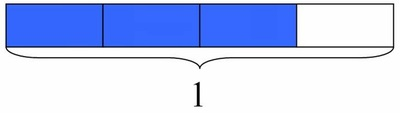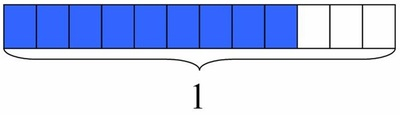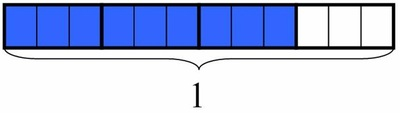# Explaining Fraction Equivalence with Pictures

Alignments to Content Standards: 4.NF.A.1

1. The rectangle below has length 1. What fraction does the shaded part represent?2. The rectangle below has the same length as the rectangle above. What fraction does the shaded part represent?3. Use the pictures to explain why the two fractions represented above are equivalent.

## IM Commentary

The purpose of this task is to provide students with an opportunity to explain fraction equivalence through visual models in a particular example. Part (c) should be approached as a discussion before students are asked to write an explanation. Students can talk generally about the relationship between the pictures ("Each of the larger pieces is broken up into 3 little pieces"), which can then be refined and connected to the appropriate operations ("There are three times as many smaller pieces as bigger pieces"). Students will need more opportunities to think about fraction equivalence with different examples and models, but this task represents a good first step.

## Attached Resources

• Lesson Plan - Comparing Fractions 1
• Explore Lesson Plan
• ## Solution

1. $\frac{3}{4}$
2. $\frac{9}{12}$
3. Three pieces in the bottom rectangle have the same size as 1 piece in the top rectangle. We can even show this by darkening the lines around groups of three small pieces in the rectangle that represents $\frac{9}{12}$:When we make groups of three in the bottom rectangle, there are 3 groups of 3 shaded pieces and 4 groups of 3 in the whole rectangle. Using these groups, we see that
\begin{align} \frac{9}{12} =& \frac{(3 \times 3)}{(4 \times 3)} \\ =& \frac{3}{4} \end{align}
of the bottom rectangle is shaded. Since the shaded portion is the same in each case but we just look at it in a different way and describe it with a different fraction, the fractions are equal. So $$\frac{9}{12} = \frac{3}{4}$$